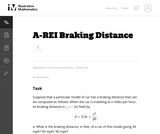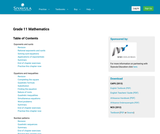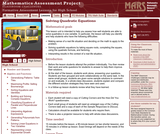# 3 Results

View
Selected filters:Conditions of Use:
No Strings Attached
Rating

This task provides an exploration of a quadratic equation by descriptive, numerical, graphical, and algebraic techniques. Based on its real-world applicability, teachers could use the task as a way to introduce and motivate algebraic techniques like completing the square, en route to a derivation of the quadratic formula.

Material Type:
Activity/Lab
Provider:
Illustrative Mathematics
Provider Set:
Illustrative Mathematics
Author:
Illustrative Mathematics
05/01/2012Rating

Material Type:
Textbook
Provider:
Siyavula
04/12/2012Rating

This lesson unit is intended to help teachers assess how well students are able to solve quadratics in one variable. In particular, the lesson will help teachers identify and help students who have the following difficulties: making sense of a real life situation and deciding on the math to apply to the problem; solving quadratic equations by taking square roots, completing the square, using the quadratic formula, and factoring; and interpreting results in the context of a real life situation.

نوع المادة:
التقييم
Lesson Plan
Provider:
Shell Center for Mathematical Education
U.C. Berkeley
Provider Set:
Mathematics Assessment Project (MAP)